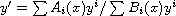Electron. J. Diff. Equ., Vol. 2011 (2011), No. 121, pp. 1-16.

### Computation of rational solutions for a first-order nonlinear differential equation Djilali Behloul, Sui Sun Cheng

Abstract:
In this article, we study differential equations of the formwhich can be elliptic, hyperbolic, parabolic, Riccati, or quasi-linear. We show how rational solutions can be computed in a systematic manner. Such results are most likely to find applications in the theory of limit cycles as indicated by Gine et al .

Submitted August 23, 2011. Published September 19, 2011.
Math Subject Classifications: 34A05.
Key Words: Polynomial solution; rational solution; nonlinear differential equation.

Show me the PDF file (261 KB), TEX file, and other files for this article.Djilali Behloul Faculté Génie Electrique Département informatique, USTHB, BP32 El Alia, Bab Ezzouar, 16111, Algiers, Algeria email: dbehloul@yahoo.fr Sui Sun Cheng Department of Mathematics, Hua University Hsinchu 30043, Taiwan email: sscheng@math.nthu.edu.tw